# bar

•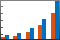## Syntax

``bar(y)``
``bar(x,y)``
``bar(___,width)``
``bar(___,style)``
``bar(___,color)``
``bar(___,Name,Value)``
``bar(ax,___)``
``b = bar(___)``

## Description

example

````bar(y)` creates a bar graph with one bar for each element in `y`. To plot a single series of bars, specify `y` as a vector of length m. The bars are positioned from `1` to m along the x-axis.To plot multiple series of bars, specify `y` as a matrix with one column for each series. ```

example

````bar(x,y)` draws the bars at the locations specified by `x`.```

example

````bar(___,width)` sets the relative bar width, which controls the separation of bars within a group. Specify `width` as a scalar value. Use this option with any of the input argument combinations in the previous syntaxes.```

example

````bar(___,style)` specifies the style of the bar groups. For example, use `'stacked'` to display each group as one multicolored bar.```

example

````bar(___,color)` sets the color for all the bars. For example, use `'r'` for red bars.```

example

````bar(___,Name,Value)` specifies properties of the bar graph using one or more name-value pair arguments. Only bar graphs that use the default `'grouped'` or `'stacked'` style support setting bar properties. Specify the name-value pair arguments after all other input arguments. For a list of properties, see Bar Properties.```

example

````bar(ax,___)` plots into the axes specified by `ax` instead of into the current axes (`gca`). The option `ax` can precede any of the input argument combinations in the previous syntaxes.```

example

````b = bar(___)` returns one or more `Bar` objects. If `y` is a vector, then `bar` creates one `Bar` object. If `y` is a matrix, then `bar` returns a `Bar` object for each series. Use `b` to set properties of the bars after displaying the bar graph.```

## Examples

collapse all

```y = [75 91 105 123.5 131 150 179 203 226 249 281.5]; bar(y)```Specify the bar locations along the x-axis.

```x = 1900:10:2000; y = [75 91 105 123.5 131 150 179 203 226 249 281.5]; bar(x,y)```Since R2023b

Create a string vector `x` containing the names of four bars. Create a numeric vector `y` containing the lengths of the bars. Then create a bar chart of `x` and `y`.

```x = ["Spring" "Summer" "Autumn" "Winter"]; y = [1 2 3 4]; bar(x,y)```Set the width of each bar to 40 percent of the total space available for each bar.

```y = [75 91 105 123.5 131 150 179 203 226 249 281.5]; bar(y,0.4)```Display four groups of three bars.

```y = [2 2 3; 2 5 6; 2 8 9; 2 11 12]; bar(y)```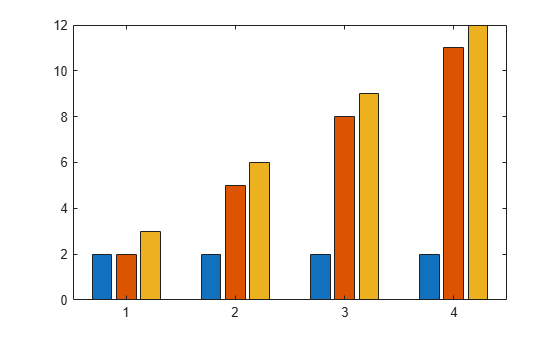Display one bar for each row of the matrix. The height of each bar is the sum of the elements in the row.

```y = [2 2 3; 2 5 6; 2 8 9; 2 11 12]; bar(y,'stacked')```Create a scalar `x` and a vector `y`. Display one stacked bar centered at `x=2020`. Each section in the stack corresponds to an element of `y`.

``` x = 2020; y = [30 50 23]; b = bar(x,y,"stacked");```Adjust the width of the stacked bar. Because the individual bars are stacked, changing the width of one `Bar` object changes all of them.

` b(1).BarWidth = 0.25;`Define `x` as a vector of three year values. Define `y` as a matrix that contains a combination of negative and positive values. Display the values in a bar graph.

```x = [1980 1990 2000]; y = [15 20 -5; 10 -17 21; -10 5 15]; bar(x,y,'stacked')```One way to indicate categories for your bars is to specify `X` as a categorical array. The `bar` function uses a sorted list of the categories, so the bars might display in a different order than you expect. To preserve the order, call the `reordercats` function.

Define `X` as categorical array, and call the `reordercats` function to specify the order for the bars. Then define `Y` as a vector of bar heights and display the bar graph.

```X = categorical({'Small','Medium','Large','Extra Large'}); X = reordercats(X,{'Small','Medium','Large','Extra Large'}); Y = [10 21 33 52]; bar(X,Y)```Define `vals` as a matrix containing the values of two data sets. Display the values in a bar graph and specify an output argument. Since there are two data sets, `bar` returns a vector containing two `Bar` objects.

```x = [1 2 3]; vals = [2 3 6; 11 23 26]; b = bar(x,vals);```Display the values at the tips of the first series of bars. Get the coordinates of the tips of the bars by getting the `XEndPoints` and `YEndPoints` properties of the first `Bar` object. Pass those coordinates to the `text` function, and specify the vertical and horizontal alignment so that the values are centered above the tips of the bars.

```xtips1 = b(1).XEndPoints; ytips1 = b(1).YEndPoints; labels1 = string(b(1).YData); text(xtips1,ytips1,labels1,'HorizontalAlignment','center',... 'VerticalAlignment','bottom')```Next, display the values above the tips of the second series of bars.

```xtips2 = b(2).XEndPoints; ytips2 = b(2).YEndPoints; labels2 = string(b(2).YData); text(xtips2,ytips2,labels2,'HorizontalAlignment','center',... 'VerticalAlignment','bottom')```Since R2019b

You can display a tiling of bar graphs using the `tiledlayout` and `nexttile` functions. Call the `tiledlayout` function to create a 2-by-1 tiled chart layout. Call the `nexttile` function to create the axes objects `ax1` and `ax2`. Display a bar graph in the top axes. In the bottom axes, display a stacked bar graph of the same data.

```y = [1 2 3; 4 5 6]; tiledlayout(2,1) % Top bar graph ax1 = nexttile; bar(ax1,y) % Bottom bar graph ax2 = nexttile; bar(ax2,y,'stacked')```Create a bar graph using red bars.

```y = [75 91 105 123.5 131 150 179 203 226 249 281.5]; bar(y,'r')```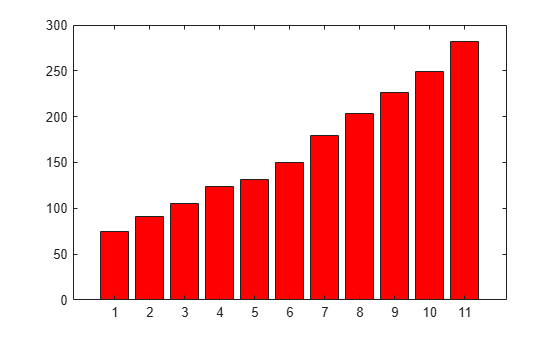Set the bar interior color and outline color using RGB triplets. Set the width of the bar outline.

```y = [75 91 105 123.5 131 150 179 203 226 249 281.5]; bar(y,'FaceColor',[0 .5 .5],'EdgeColor',[0 .9 .9],'LineWidth',1.5)```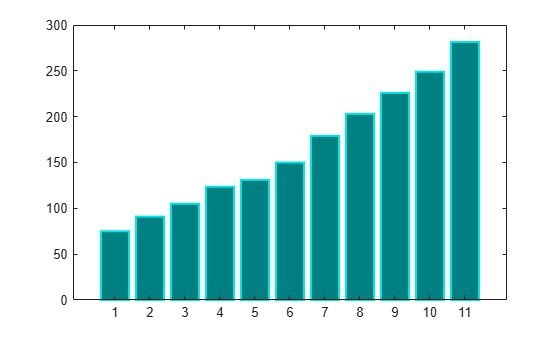Control individual bar colors using the `CData` property of the `Bar` object.

Create a bar chart and assign the `Bar` object to a variable. Set the `FaceColor` property of the `Bar` object to `'flat'` so that the chart uses the colors defined in the `CData` property. By default, the `CData` property is prepopulated with a matrix of the default RGB color values. To change a particular color, change the corresponding row in the matrix. For example, change the color of the second bar.

```b = bar(rand(10,1)); b.FaceColor = 'flat'; b.CData(2,:) = [.5 0 .5];```Create a bar chart that uses colormap colors by setting the `FaceColor` property to `'flat'`. Then set the `CData` property for each `Bar` object to an integer.

```y = [1 3 5; 3 2 7; 3 4 2]; b = bar(y,'FaceColor','flat'); for k = 1:size(y,2) b(k).CData = k; end```Since R2023b

Named color palettes provide a convenient way to change the colors of a chart. This example compares a bar chart with three different color palettes.

Create a bar chart of random numbers using the default palette.

`bar(rand(3,5))`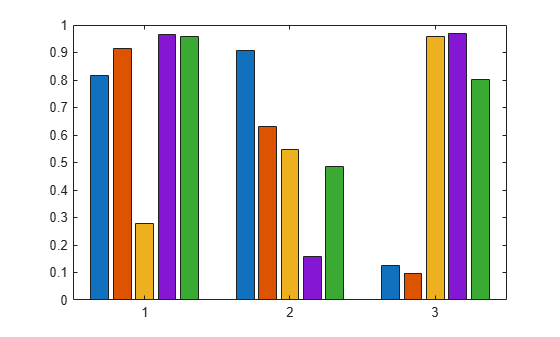Change the color palette to `reef` by using the `colororder` function.

`colororder("reef")`Change the color palette to `earth`.

`colororder("earth")`Create matrix `y`, where each column is a series of data. Call the `bar` function to display the data in a bar graph, and specify an output argument. The output is a vector of three `Bar` objects, where each object corresponds to a different series. This is true whether the bars are grouped or stacked.

```y = [10 15 20; 30 35 40; 50 55 62]; b = bar(y);```Make the third series of bars green.

`b(3).FaceColor = [.2 .6 .5];`## Input Arguments

collapse all

x-coordinates, specified as a scalar, vector, matrix, string array, or cell array of character vectors. The values of `x` do not need to be in order, but the size of `x` depends on the size of `y` and how you want to display your data. This table describes the most common situations.

PresentationHow to Specify X and YExample
Display one series of bars.

Specify `x` and `y` as vectors that are the same length. The values in `x` must be unique, but the values in `y` do not need to be unique.

```x = [1980 1990 2000]; y = [10 20 30]; bar(x,y)```Display multiple series of bars in groups.

Specify either of these combinations:

• Specify `x` and `y` as matrices of equal size. Each column of `y` corresponds to a series of bars. By default, each series is a different color. To ensure consistent placement of the groups, specify the columns of `x` as identical vectors. The values within a column must be unique, even though the columns are repeated.

• Specify `x` as a vector of unique values, and specify `y` as a matrix. The length of `x` must equal the length of at least one dimension of `y`. The other dimension of `y` contains values for the different series of bars.

```x = [1980 1980 1980 1990 1990 1990]; y = [2 6 9 11 22 32]; bar(x,y)```
Or
```x = [1980 1990]; y = [2 6 9 11 22 32]; bar(x,y)```Display one group of bars centered at one `x` value.

Specify `x` as a scalar and `y` as a vector.

```x = 1990; y = [10 20 30]; bar(x,y)```Data Types: `single` | `double` | `int8` | `int16` | `int32` | `int64` | `uint8` | `uint16` | `uint32` | `uint64` | `categorical` | `datetime` | `duration` | `string` (since R2023b) | `cell` (since R2023b)

y-coordinates, specified as a scalar, vector, or matrix. The size of `y` depends on the size of `x` and how you want to display your data. This table describes the most common situations.

PresentationHow to Specify X and YExample
Display one series of bars.

Specify `x` and `y` as vectors that are the same length. The values in `x` must be unique, but the values in `y` do not need to be unique.

```x = [1980 1990 2000]; y = [10 20 30]; bar(x,y)```Display multiple series of bars in groups.

Specify either of these combinations:

• Specify `x` and `y` as matrices of equal size. Each column of `y` corresponds to a series of bars. By default, each series is a different color. To ensure consistent placement of the groups, specify the columns of `x` as identical vectors. The values within a column must be unique, even though the columns are repeated.

• Specify `x` as a vector of unique values, and specify `y` as a matrix. The length of `x` must equal the length of at least one dimension of `y`. The other dimension of `y` contains values for the different series of bars.

```x = [1980 1980 1980 1990 1990 1990]; y = [2 6 9 11 22 32]; bar(x,y)```
Or
```x = [1980 1990]; y = [2 6 9 11 22 32]; bar(x,y)```Display one group of bars centered at one `x` value.

Specify `x` as a scalar and `y` as a vector.

```x = 1990; y = [10 20 30]; bar(x,y)```Data Types: `single` | `double` | `int8` | `int16` | `int32` | `int64` | `uint8` | `uint16` | `uint32` | `uint64` | `duration`

Bar width, specified as a fraction of the total space available for each bar. The default of `0.8` means the bar width is 80% of the space from the previous bar to the next bar, with 10% of that space on each side.

If the width is `1`, then the bars within a group touch one another.

Example: `bar([1 2 3],0.5)` creates bars that use 50% of the available space.

Data Types: `single` | `double` | `int8` | `int16` | `int32` | `int64` | `uint8` | `uint16` | `uint32` | `uint64`

Group style, specified by one of these values.

StyleResultExample

`'grouped'`

Display each group as adjacent bars that are centered around their corresponding `x` value.`'stacked'`

Display each group as one multicolored bar. The length of a bar is the sum of the elements in the group.

If `y` is a vector, then the result is the same as `'grouped'`.`'histc'`

Display the bars in histogram format, in which the bars in a group touch one another. The trailing edge of each group is aligned with the corresponding `x` value.

Note

A better way to display a histogram is to call the `histogram` function.`'hist'`

Display the bars in histogram format. Each group is centered at the corresponding `x` value.

Note

A better way to display a histogram is to call the `histogram` function.Bar color, specified as one of the options in this table.

Color NameShort NameAppearance
`'red'``'r'``'green'``'g'``'blue'``'b'``'cyan'` `'c'``'magenta'``'m'``'yellow'``'y'``'black'``'k'``'white'``'w'`Axes object. If you do not specify an axes, then `bar` uses the current axes for the bar graph.

### Name-Value Arguments

Specify optional pairs of arguments as `Name1=Value1,...,NameN=ValueN`, where `Name` is the argument name and `Value` is the corresponding value. Name-value arguments must appear after other arguments, but the order of the pairs does not matter.

Before R2021a, use commas to separate each name and value, and enclose `Name` in quotes.

Example: `bar([10 20 30],'EdgeColor','g')` specifies a green outline around the bars.

The `Bar` properties listed here are only a subset. For a complete list, see Bar Properties.

Note

• The properties listed here are only a subset. For a complete list, see Bar Properties.

• You can set these properties only on bar graphs that use the default `'grouped'` or `'stacked'` style.

Outline color, specified as `'flat'`, an RGB triplet, a hexadecimal color code, a color name, or a short name. If there are 150 bars or fewer, the default value is `[0 0 0]`, which corresponds to black. If there are more than 150 adjacent bars, the default value is `'none'`.

Starting in R2017b, the `'flat'` option uses the `CData` values to color the edges. In previous releases, the `'flat'` option colored the edges using colors from the colormap.

For a custom color, specify an RGB triplet or a hexadecimal color code.

• An RGB triplet is a three-element row vector whose elements specify the intensities of the red, green, and blue components of the color. The intensities must be in the range `[0,1]`, for example, ```[0.4 0.6 0.7]```.

• A hexadecimal color code is a string scalar or character vector that starts with a hash symbol (`#`) followed by three or six hexadecimal digits, which can range from `0` to `F`. The values are not case sensitive. Therefore, the color codes `"#FF8800"`, `"#ff8800"`, `"#F80"`, and `"#f80"` are equivalent.

Alternatively, you can specify some common colors by name. This table lists the named color options, the equivalent RGB triplets, and hexadecimal color codes.

Color NameShort NameRGB TripletHexadecimal Color CodeAppearance
`"red"``"r"``[1 0 0]``"#FF0000"``"green"``"g"``[0 1 0]``"#00FF00"``"blue"``"b"``[0 0 1]``"#0000FF"``"cyan"` `"c"``[0 1 1]``"#00FFFF"``"magenta"``"m"``[1 0 1]``"#FF00FF"``"yellow"``"y"``[1 1 0]``"#FFFF00"``"black"``"k"``[0 0 0]``"#000000"``"white"``"w"``[1 1 1]``"#FFFFFF"``"none"`Not applicableNot applicableNot applicableNo color

Here are the RGB triplets and hexadecimal color codes for the default colors MATLAB® uses in many types of plots.

`[0 0.4470 0.7410]``"#0072BD"``[0.8500 0.3250 0.0980]``"#D95319"``[0.9290 0.6940 0.1250]``"#EDB120"``[0.4940 0.1840 0.5560]``"#7E2F8E"``[0.4660 0.6740 0.1880]``"#77AC30"``[0.3010 0.7450 0.9330]``"#4DBEEE"``[0.6350 0.0780 0.1840]``"#A2142F"`Example: `b = bar(1:10,'EdgeColor','red')`

Example: `b.EdgeColor = [0 0.5 0.5];`

Example: `b.EdgeColor = 'flat';`

Example: `b.EdgeColor = '#D2F9A7';`

Fill color, specified as `'flat'`, an RGB triplet, a hexadecimal color code, a color name, or a short name. The `'flat'` option uses the `CData` property value of the `Bar` object to color the faces.

For a custom color, specify an RGB triplet or a hexadecimal color code.

• An RGB triplet is a three-element row vector whose elements specify the intensities of the red, green, and blue components of the color. The intensities must be in the range `[0,1]`, for example, ```[0.4 0.6 0.7]```.

• A hexadecimal color code is a string scalar or character vector that starts with a hash symbol (`#`) followed by three or six hexadecimal digits, which can range from `0` to `F`. The values are not case sensitive. Therefore, the color codes `"#FF8800"`, `"#ff8800"`, `"#F80"`, and `"#f80"` are equivalent.

Alternatively, you can specify some common colors by name. This table lists the named color options, the equivalent RGB triplets, and hexadecimal color codes.

Color NameShort NameRGB TripletHexadecimal Color CodeAppearance
`"red"``"r"``[1 0 0]``"#FF0000"``"green"``"g"``[0 1 0]``"#00FF00"``"blue"``"b"``[0 0 1]``"#0000FF"``"cyan"` `"c"``[0 1 1]``"#00FFFF"``"magenta"``"m"``[1 0 1]``"#FF00FF"``"yellow"``"y"``[1 1 0]``"#FFFF00"``"black"``"k"``[0 0 0]``"#000000"``"white"``"w"``[1 1 1]``"#FFFFFF"``"none"`Not applicableNot applicableNot applicableNo color

Here are the RGB triplets and hexadecimal color codes for the default colors MATLAB uses in many types of plots.

`[0 0.4470 0.7410]``"#0072BD"``[0.8500 0.3250 0.0980]``"#D95319"``[0.9290 0.6940 0.1250]``"#EDB120"``[0.4940 0.1840 0.5560]``"#7E2F8E"``[0.4660 0.6740 0.1880]``"#77AC30"``[0.3010 0.7450 0.9330]``"#4DBEEE"``[0.6350 0.0780 0.1840]``"#A2142F"`Starting in R2017b, the default value is an RGB triplet from the `ColorOrder` property of the axes. In previous releases, the default value was `'flat'` and the colors were based on the colormap.

Example: `b = bar(1:10,'FaceColor','red')`

Example: `b.FaceColor = [0 0.5 0.5];`

Example: `b.FaceColor = 'flat';`

Example: `b.FaceColor = '#D2F9A7';`

Color data, specified as one of these values:

• RGB triplet — Single RGB color value applies to all bars.

• Three-column matrix — One color per bar. Each row in the matrix specifies an RGB triplet for a particular bar.

• Scalar — Single color applies to all bars, where the color comes from the colormap.

• Vector — One color per bar. The colors come from the colormap.

By default, when you create a bar chart, the `CData` property contains a three-column matrix of RGB triplets. You can change the color for a particular bar by changing the corresponding row in the matrix.

This property applies only when the `FaceColor` or `EdgeColor` property is set to `'flat'`.

#### Example

Change the color for a particular bar by setting the `FaceColor` property to `'flat'`. Then change the corresponding row in the `CData` matrix to the new RGB triplet. For example, change the color of the second bar.

```b = bar(1:10,'FaceColor','flat'); b.CData(2,:) = [0 0.8 0.8];```Baseline value, specified as a numeric scalar value.

The baseline value that you specify applies to either the x-axis or the y-axis depending on the bar chart orientation. If you change the orientation of the bar chart from vertical to horizontal, or vice versa, the baseline value might change. Set the `BaseValue` property after setting the `Horizontal` property.

Line style of bar outlines, specified as one of the line styles in this table.

Line StyleDescriptionResulting Line
`"-"`Solid line`"--"`Dashed line`":"`Dotted line`"-."`Dash-dotted line`"none"`No lineNo line

Width of bar outlines, specified as a positive value in point units. One point equals 1/72 inch.

Example: `1.5`

Data Types: `single` | `double` | `int8` | `int16` | `int32` | `int64` | `uint8` | `uint16` | `uint32` | `uint64`

## Output Arguments

collapse all

`Bar` objects. Use the elements in `b` to access and modify properties of a specific `Bar` object after it has been created. The number of `Bar` objects depends on the size of `y`. If `y` is a vector, then `b` is one `Bar` object. If `y` is a matrix, then `b` is a vector containing a `Bar` object for each series in `y`.

collapse all

### Series of Bars

A series consists of the bars at all locations in `X` for a particular set of data. By default, each series of bars is indicated by a different color.

### Group of Bars

A group consists of all the bars at a particular location in `X`.

## Version History

Introduced before R2006a

expand all